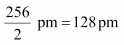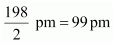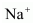• +91 9971497814
• info@interviewmaterial.com

# Chapter 3- Classification of Elements and Periodicity in Properties Interview Questions Answers

### Related Subjects

Question 1 : What is the basic theme of organization in the periodic table?

Answer 1 : It is to characterize the elements in periods and groups as per their properties. So, this course of action makes the investigation of elements and compounds of elements in a simple and methodical way. In this periodic table, elements with comparative properties are set in a similar group.

Question 2 : Which important property did Mendeleev use to classify the elements in his periodic table and did he stick to that?

Mendeleev organised the components in his periodic table, according to the order of their atomic weight. Mendeleev organized the components in groups and periods according to the increasing atomic weight. Mendeleev set the elements which are having comparative properties in similar groups.

Nonetheless, he didn’t adhere to an arrangement that he gave for long. He discovered that if the elements were organized according to their increasing atomic weights, then a few elements did not match within this plan of characterization.

In this manner, he overlooked the order of atomic weights now and again. For instance, the atomic mass of iodine is lower than the atomic mass of tellurium.

Still, Mendeleev set tellurium (in Group 6) ahead of iodine (in Group 7) essentially in light of the fact that iodine’s properties are so comparable to fluorine, chlorine, and bromine.

Question 3 : What is the basic difference in approach between the Mendeleev’s Periodic Law and the Modern Periodic Law?

 Mendeleev’s Approach for periodic law Modern approach for the periodic law Chemical properties and physical properties of the elements are the periodic functions of the atomic mass of the corresponding elements. Chemical properties and physical properties of the elements are the periodic functions of the atomic numbers of the corresponding elements.

Question 4 : On the basis of quantum numbers, justify that the sixth period of the periodic table should have 32 elements.

In a periodic table containing elements, a period shows the value of a principal quantum number (n) for the furthest shells. Every period starts with the filling with the principal quantum number (n). And n’s value for the 6th period is equal to 6. Now, for n = 6, the azimuthal quantum number (l) can have “0, 1, 2, 3, 4” values.

As indicated by Aufbau’s rule, electrons will be added to various orbitals according to their increasing energies. Here, the 6d subshell is having much higher energy than the energy of 7s subshell.

In the sixth period, the electrons can occupy in just 6s, 4f, 5d, and 6 p subshells. 6s is having 1 orbital, 4f is having 7 orbitals, 5d is having 5 orbitals, and 6p is having 3 orbitals. In this way, there are a sum of 16 (1 + 7 + 5 + 3 = 16) orbitals accessible. As indicated by Pauli’s exclusion, one orbital can only accommodate at max 2 electrons.

Hence, sixteen orbitals can have 32 electrons.

Subsequently, the 6th period of the period table ought to have 32 elements.

Question 5 : In terms of period and group where would you locate the element with Z =114?

Elements whose atomic number is from Z = 87 to Z = 114 are available in the seventh period of the periodic table. Therefore, the element having Z = 114 is available in the seventh period in the periodic table.

In the seventh period, initial 2 elements with Z = 87 and Z= 88 are the elements of s-block and the following 14 elements except Z = 89 i.e., those from Z = 90 to Z = 103 are elements of f – block, and next 10 elements from Z = 89 and Z = 104 to Z = 112 are elements of d-block, next the elements from Z = 113 to Z = 118 are elements of p-block. In this manner, the element Z = 114 is the 2nd element of p-block in the seventh period of the periodic table.

Therefore, the element Z = 114 is available in the seventh period and fourth group in the periodic table.

Question 6 :
What is the atomic number of element keeping in mind both the cases given below;

1. Element is in 3rd period of the periodic table.
2. Element is in 17th group of the periodic table.

Answer 6 : The first period is having 2 elements and the second period is having 8 elements. So, the third period begins with element Z = 11. Presently, the third period contains 8 elements. So, the 18th element is the last element of the third period and this 18th element is present in the 18th group. Thus, the element in the seventeenth group of the 3rd period is having atomic number 17 i.e. Z = 17.

Question 7 :
Which element do you think would have been named by
(i) Lawrence Berkeley Laboratory
(ii) Seaborg’s group?

(i) Lawrencium (Lr) which has an atomic number, Z = 103 and Berkelium (Bk) which has an atomic number, Z = 97

(ii) Seaborgium (Sg) which has an atomic number, Z = 106

Question 8 : Why do elements in the same group have similar physical and chemical properties?

Answer 8 : The chemical and physical properties of any elements rely on the number of valence electrons. In periodic table elements are in the same group are having the same quantity of valence electrons. This is why elements present in the same group are having similar chemical and physical properties.

Question 9 : What does atomic radius and ionic radius really mean to you?

Atomic radius is the radius of an atom. It measures the size of an atom. If the element is a metal, then the atomic radius refers to the metallic radius, and if the element is a non-metal, then it refers to the covalent radius. Metallic radius is calculated as half the internuclear distance separating the metal cores in the metallic crystal. For example, the internuclear distance between two adjacent copper atoms in solid copper is 256 pm. Thus, the metallic radius of copper is taken as.Covalent radius is measured as the distance between two atoms when they are found together by a single bond in a covalent molecule. For example, the distance between two chlorine atoms in chlorine molecule is 198 pm. Thus, the covalent radius of chlorine is taken as .Ionic radius means the radius of an ion (cation or anion). The ionic radii can be calculated by measuring the distances between the cations and anions in ionic crystals.

Since a cation is formed by removing an electron from an atom, the cation has fewer electrons than the parent atom resulting in an increase in the effective nuclear charge. Thus, a cation is smaller than the parent atom. For example, the ionic radius ofion is 95 pm, whereas the atomic radius of Na atom is 186 pm. On the other hand, an anion is larger in size than its parent atom. This is because an anion has the same nuclear charge, but more electrons than the parent atom resulting in an increased repulsion among the electrons and a decrease in the effective nuclear charge. For example, the ionic radius of F– ion is 136 pm, whereas the atomic radius of F atom is 64 pm.

Question 10 : How do atomic radius vary in a period and in a group? How do you explain the variation?

Atomic radius declines as we move from left to right in a period. It happens because in a period, the external electrons are available in a similar valence shell so, the atomic number increments from left to right in a period, which results in an increase in the effective nuclear charge. Therefore, the attraction of electrons towards the nucleus is increased.

Also, atomic radius declines as we move from top to bottom in the group. It happens because as we move down in a group then there is an increase in principal quantum number(n) which brings about an increase in the distance between the nucleus and the valence electrons.

Todays Deals### Chapter 3- Classification of Elements and Periodicity in Properties Contributorskrishan

Name:
Email:

# Latest News# 9000 interview questions in different categories